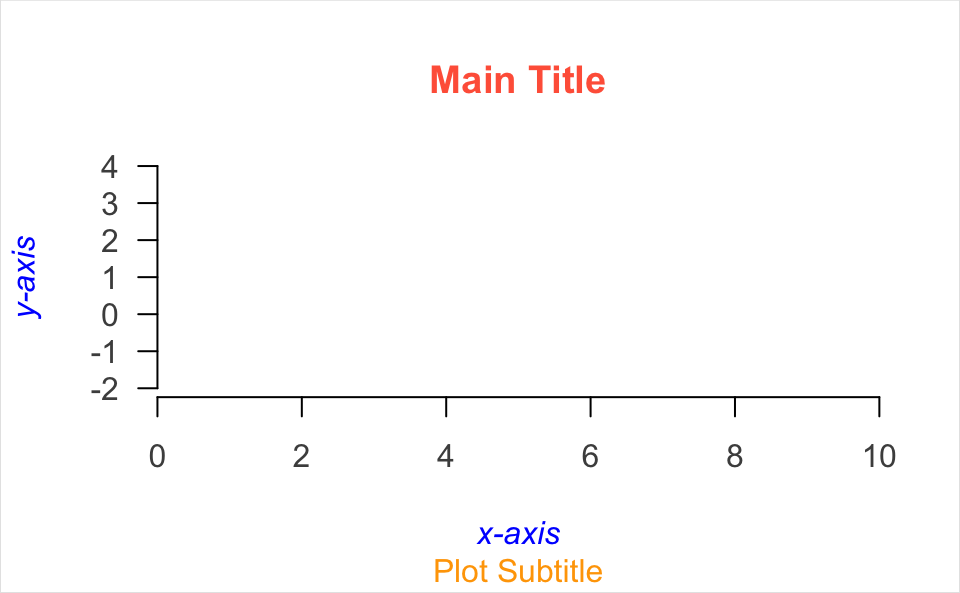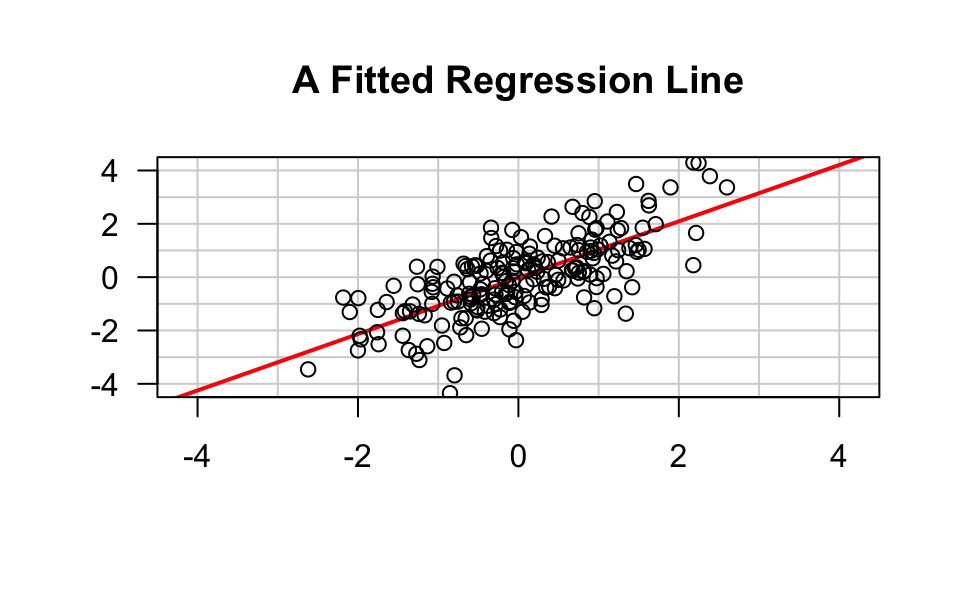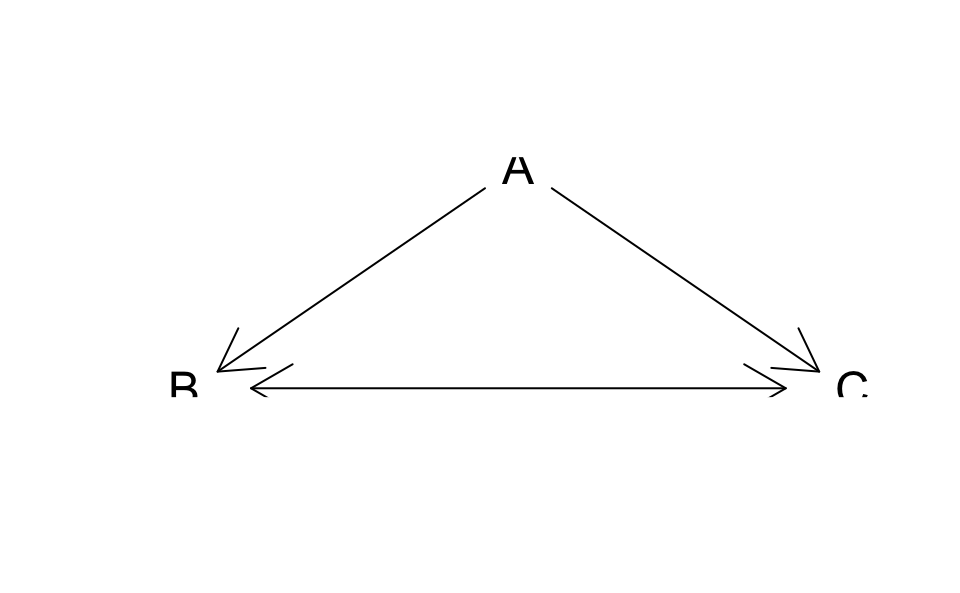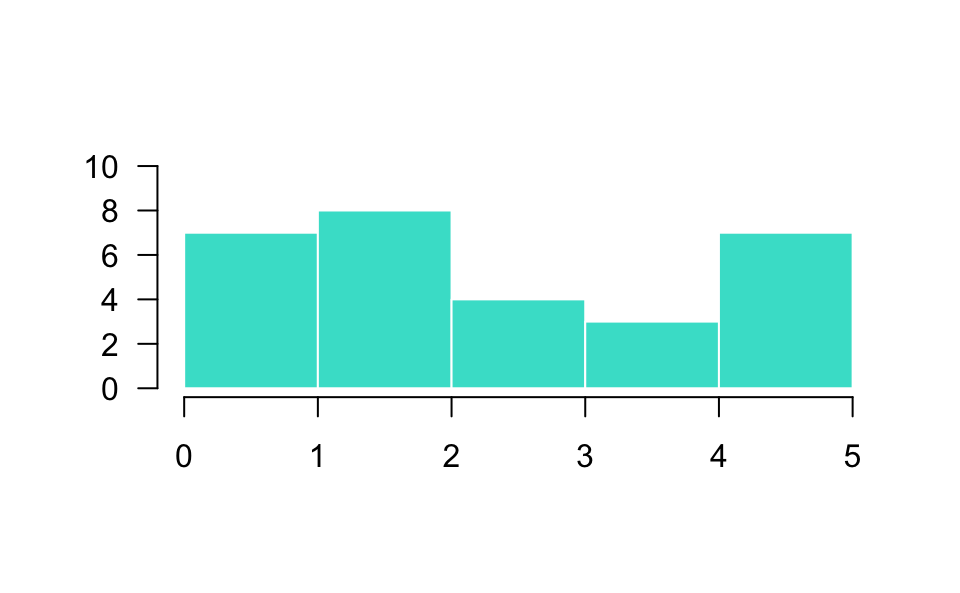# 12 Plots from Scratch

In this chapter we describe one of my favorite graphing approaches to create what I like to call plots from scratch. That is, making a graph in a way that we have full control of all the nitty-gritty details of a plot.

## 12.1 Making a graphic from scratch

In addition to using plot(), possibly calling one or more low-level functions, you should know that it is also possible to create a plot from scratch. Although this procedure is less documented, it is extremely flexible and powerful. The general recipe to create a plot with this approach is as follows:

• call plot.new() to start a new plot frame

• call plot.window() to define coordinates

• then call low-level functions:

• typical options involve axis()

• then title() (title, subtitle)

• after that call other function: e.g. points(), lines(), etc

plot.new()
plot.window(xlim = c(0, 10), ylim = c(-2, 4), xaxs = "i")
axis(side = 1, col.axis = "grey30")
axis(side = 2, col.axis = "grey30", las = 1)
title(main = "Main Title",
col.main = "tomato",
sub = "Plot Subtitle",
col.sub = "orange",
xlab = "x-axis",
ylab = "y-axis",
col.lab = "blue",
font.lab = 3)
box("figure", col = "grey90")Here’s another example:

set.seed(5)
x <- rnorm(200)
y <- x + rnorm(200)

plot.new()
plot.window(xlim = c(-4.5, 4.5), xaxs = "i",
ylim = c(-4.5, 4.5), yaxs = "i")
z <- lm(y ~ x)
abline(h = -4:4, v = -4:4, col = "lightgrey")
abline(a = coef(z), b = coef(z), lwd = 2, col = "red")
points(x, y)
axis(side = 1)
axis(side = 2, las = 1)
box()
title(main = "A Fitted Regression Line")### 12.1.1 How does it work

You start a new plot with plot.new()

• plot.new() opens a new (empty) plot frame

• plot.new() chooses a default plotting region

After starting with plot.new(), use plot.window() to set up the coordinate system for the plotting frame

# axis limits (0,1)x(0,1)
plot.window(xlim = c(0, 1), ylim = c(0, 1))

By default plot.window() produces axis limits which are expanded by 6% over those actually specified.

The default limits expansion can be turned-off by specifying xaxs = "i" and/or yaxs = "i"

plot.window(xlim, ylim, xaxs = "i")

#### Aspect Ratio Control

Another important argument is asp, which allows us to specify the aspect ratio

plot.window(xlim, ylim, xaxs = "i", asp = 1)

asp = 1 means that unit steps in the x and y directions produce equal distances in the x and y directions on the plot.

(Important for avoiding distortion of circles that look like ellipses)

#### Drawing Axes

The axis() function can be used to draw axes at any of the four sides of a plot.

• side = 1 below the graph

• side = 2 to the left of the graph

• side = 3 above the graph

• side = 4 to the right of the graph

Axes can be customized via several arguments (see ?axis)

• location of tick-marks

• labels of axis

• colors

• sizes

• text fonts

• text orientation

#### Plot Annotatiosn

The function title() allows us to include labels in the margins

• main main title above the graph

• sub subtitle below the graph

• xlab label for the x-axis

• ylab label for the y-axis

The annotations can be customized with additional arguments for the fonts, colors, and size (expansion)

• font.main, col.main, cex.main

• font.sub, col.sub, cex.sub

• font.lab, col.lab, cex.lab

### 12.1.2 Drawing Arrows

plot.new()
plot.window(xlim = c(0, 1), ylim = c(0, 1))
arrows(0.05, 0.075, 0.45, 0.9, code = 1)
arrows(0.55, 0.9, 0.95, 0.075, code = 2)
arrows(0.1, 0, 0.9, 0, code = 3)
text(0.5, 1, "A", cex = 1.5)
text(0, 0, "B", cex = 1.5)
text(1, 0, "C", cex = 1.5)### 12.1.3 Drawing Rectangles

Rectangles can be drawn with the function:

rect(x0, y0, x1, y1, col = str, border = str)
• x0, y0, x1, y1 give the coordinates of diagonally opposite corners of the rectangles/

• col specifies the color of the interior.

• border specifies the color of the border/

# barplot "manually" constructed
plot.new()
plot.window(xlim = c(0, 5), ylim = c(0, 10))
rect(0:4, 0, 1:5, c(7, 8, 4, 3),
col = "turquoise",
border = "white")
axis(1)
axis(2, las = 1)